## Archive for the ‘PID’ Category

### PID: When Should I Compute the Integral Term?

Sunday, July 24th, 2011

Recently there was a suggestion posted to the Beginner’s PID Series. The contention was that if you solve things in the Laplace domain, it specifies a different way of executing the Integral Term. Rather than looking at the sum of error for THIS point, the commenter suggested, you should look at the sum from the last point.

So the current code is this:

```      /*Compute all the working error variables*/
double input = *myInput;
double error = *mySetpoint - input;
ITerm+= (ki * error);
if(ITerm > outMax) ITerm= outMax;
else if(ITerm < outMin) ITerm= outMin;
double dInput = (input - lastInput);

/*Compute PID Output*/
double output = kp * error + ITerm- kd * dInput;
```

```      /*Compute all the working error variables*/
double input = *myInput;
double error = *mySetpoint - input;

double dInput = (input - lastInput);

/*Compute PID Output*/
double output = kp * error + ITerm- kd * dInput;

ITerm+= (ki * error);
if(ITerm > outMax) ITerm= outMax;
else if(ITerm < outMin) ITerm= outMin;
```

I had never seen it done this way, but I figured I’d give it a shot. The test I devised was a simple setpoint step, followed by a ramp down.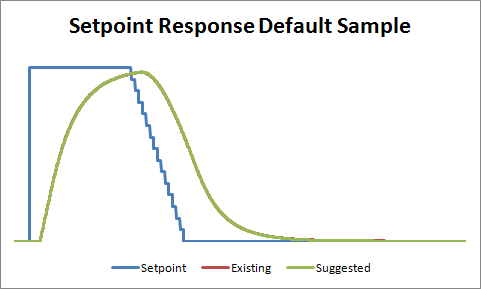With the controller set at the default sample time, the difference was imperceptible. To try and highlight the difference between the two methods, I decided to bump up the PID sample time from the default of 100mS to 5 seconds.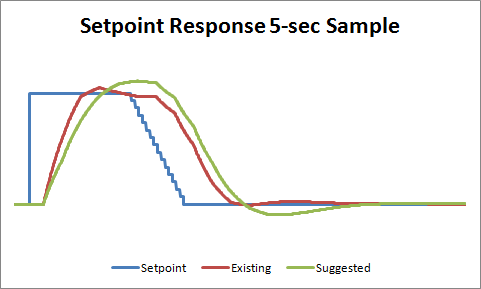Ok so here we can see a clear winner. The existing PID code performs better than suggested, probably because the integral term gets to react to process changes 5 seconds sooner. But just to make sure I wasn’t missing anything, I decided to do another test. Instead of a setpoint change, I induced a load change in the system.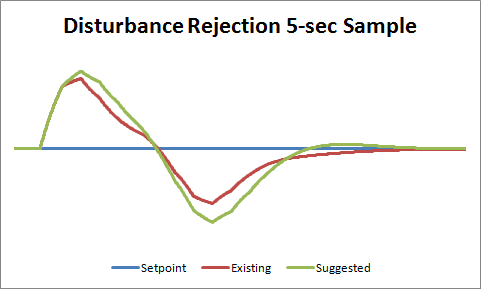Once again, the existing PID code performed better, handling the load change more quickly.

So the verdict? While this was a fun excersize, I think the results are clear. I’ll leave the code as it is.

### Reflow Oven Shield [PID Showcase]

Friday, July 22nd, 2011

The reason I created the PID library was to make it easier for people to integrate PID control into their projects. Surprisingly, it’s actually happening! I’m starting to see a lot of really cool implementations out there. As a result, I’ve decided to start a new category of post: the PID Showcase. These are projects that make me feel that all that work was worth it.

So… here’s the first one: the Reflow Oven Controller Shield from RocketScream.com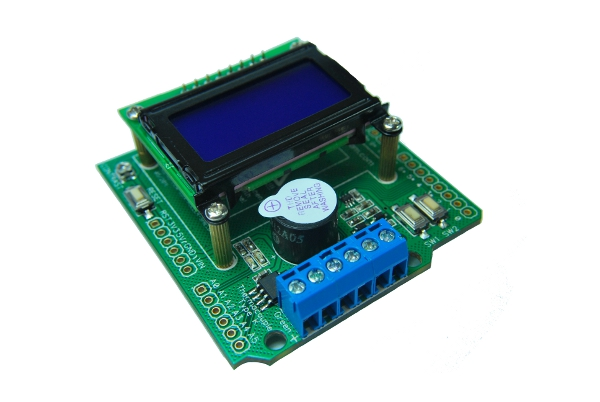It integrates all the components necessary to turn a toaster oven into a reflow oven. Thermocouple Chip… LCD… buzzer… awesome. But the part that I love is how they completely owned the PID part of this project. Look at this reflow curve:They made use of setpoint ramping to get the heating rates just right. The output is phenomenal. This came out of a toaster oven:Excellent work!

### PID Q&A Group

Monday, April 25th, 2011

Over the past week I’ve had several great conversations regarding the new PID Library, and regarding PID in general. Of course those are all in my email, so you can’t see them.

This highlights another problem with the previous version of the library. The main place for online Q&A was an unwieldy thread on the Arduino forum. You could (and can) email me of course, but that’s not how some people operate.

So I’m trying a little experiment. I’ve created a google group in an attempt to make the Q&A experience better for everyone. If you’ve got a question, shoot! If you’ve successfully implemented a PID, please share!

(if you hurry you can be the first person, IN THE WORLD, to post on the group)First-post prize goes to Eric Miller, who gets bonus points for not saying “first!”

### How Fast is the Arduino PID Library?

Wednesday, April 20th, 2011

A couple people have asked me about speed since v1 was released. Since I had no idea, I decided I should do a little benchmarking. (Tests were done on a Duemilanove with the ATMega168.)

### The Test

```#include <PID_v1.h>

double Setpoint, Input, Output;
PID myPID(&Input, &Output, &Setpoint,2,5,1, DIRECT);

void setup()
{
Setpoint = 100;

myPID.SetMode(AUTOMATIC);
Serial.begin(9600);
}

void loop()
{
unsigned long startTime = millis();
/*Do a PID calculation 10000 times*/
for(int i=0;i<10000;i++)
{
myPID.Compute();
analogWrite(3,Output);
}
/*print the elapsed time*/
Serial.println(millis()-startTime);
}
```

The code above is a modification of the Basic PID example. Rather than do the pid stuff once though, it calls it 10000 times in a row. Dividing the resulting elapsed time by 10000 gives us precision down into the microsecond range.

Not pictured in this code, I also went into PID_v1.cpp and made SampleTime=0. That ensured that every time Compute() was called, the PID equation was evaluated.

One last note. Notice that analogRead and analogWrite are in the for loop. I couldn’t think of a situation where you’d have a PID without also having those functions somewhere in the loop, so I included them in the test.

### The Results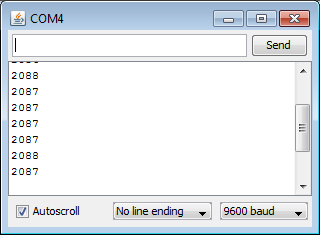2088 mSec / 10000 = 0.21 mSec
Ok. Pretty fast. Now just for fun, I commented out the read and write code (lines 21 & 23) to see how much of the 0.21 was due to the Compute function: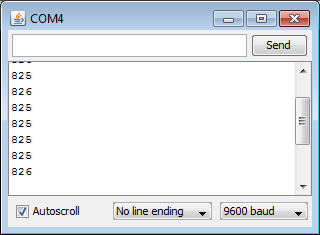826mSec / 10000 = 0.08 mSec
So the PID was only responsible for about a third of the total time. Unless I’m missing something (please tell me if I am,) it looks like the Compute function is only slightly slower than an analog read or an analog write!

### Some Closing Thoughts

Looking at these results, your first inclination might be to make the sample time as small as possible (1 mSec.) After all, that’s WAY bigger than the 0.08 mSec required. 2 things to remember:

1. There’s other code in your program. All together it may add up to more than 1mSec
2. Even if your loop time is less than 1 mSec (say it’s 0.7 mSec,) Compute only gets called once per loop, so the pid will actually be evaluated every 1.4 mSec.

Bottom line, if you’re looking to evaluate the pid really quickly, you need to be careful to avoid weird performance.

### Improving the Beginner’s PID: Direction

Friday, April 15th, 2011

(This is the last modification in a larger series on writing a solid PID algorithm)

### The Problem

The processes the PID will be connected to fall into two groups: direct acting and reverse acting. All the examples I’ve shown so far have been direct acting. That is, an increase in the output causes an increase in the input. For reverse acting processes the opposite is true. In a refrigerator for example, an increase in cooling causes the temperature to go down. To make the beginner PID work with a reverse process, the signs of kp, ki, and kd all must be negative.

This isn’t a problem per se, but the user must choose the correct sign, and make sure that all the parameters have the same sign.

### The Solution

To make the process a little simpler, I require that kp, ki, and kd all be >=0. If the user is connected to a reverse process, they specify that separately using the SetControllerDirection function. this ensures that the parameters all have the same sign, and hopefully makes things more intuitive.

### The Code

```/*working variables*/
unsigned long lastTime;
double Input, Output, Setpoint;
double ITerm, lastInput;
double kp, ki, kd;
int SampleTime = 1000; //1 sec
double outMin, outMax;
bool inAuto = false;

#define MANUAL 0
#define AUTOMATIC 1

#define DIRECT 0
#define REVERSE 1
int controllerDirection = DIRECT;

void Compute()
{
if(!inAuto) return;
unsigned long now = millis();
int timeChange = (now - lastTime);
if(timeChange>=SampleTime)
{
/*Compute all the working error variables*/
double error = Setpoint - Input;
ITerm+= (ki * error);
if(ITerm > outMax) ITerm= outMax;
else if(ITerm < outMin) ITerm= outMin;
double dInput = (Input - lastInput);

/*Compute PID Output*/
Output = kp * error + ITerm- kd * dInput;
if(Output > outMax) Output = outMax;
else if(Output < outMin) Output = outMin;

/*Remember some variables for next time*/
lastInput = Input;
lastTime = now;
}
}

void SetTunings(double Kp, double Ki, double Kd)
{
if (Kp<0 || Ki<0|| Kd<0) return;

double SampleTimeInSec = ((double)SampleTime)/1000;
kp = Kp;
ki = Ki * SampleTimeInSec;
kd = Kd / SampleTimeInSec;

if(controllerDirection ==REVERSE)
{
kp = (0 - kp);
ki = (0 - ki);
kd = (0 - kd);
}
}

void SetSampleTime(int NewSampleTime)
{
if (NewSampleTime > 0)
{
double ratio  = (double)NewSampleTime
/ (double)SampleTime;
ki *= ratio;
kd /= ratio;
SampleTime = (unsigned long)NewSampleTime;
}
}

void SetOutputLimits(double Min, double Max)
{
if(Min > Max) return;
outMin = Min;
outMax = Max;

if(Output > outMax) Output = outMax;
else if(Output < outMin) Output = outMin;

if(ITerm > outMax) ITerm= outMax;
else if(ITerm < outMin) ITerm= outMin;
}

void SetMode(int Mode)
{
bool newAuto = (Mode == AUTOMATIC);
if(newAuto == !inAuto)
{  /*we just went from manual to auto*/
Initialize();
}
inAuto = newAuto;
}

void Initialize()
{
lastInput = Input;
ITerm = Output;
if(ITerm > outMax) ITerm= outMax;
else if(ITerm < outMin) ITerm= outMin;
}

void SetControllerDirection(int Direction)
{
controllerDirection = Direction;
}
```

## PID COMPLETE

And that about wraps it up. We’ve turned “The Beginner’s PID” into the most robust controller I know how to make at this time. For those readers that were looking for a detailed explanation of the PID Library, I hope you got what you came for. For those of you writing your own PID, I hope you were able to glean a few ideas that save you some cycles down the road.

Two Final Notes:

1. If something in this series looks wrong please let me know. I may have missed something, or might just need to be clearer in my explanation. Either way I’d like to know.
2. This is just a basic PID. There are many other issues that I intentionally left out in the name of simplicity. Off the top of my head: feed forward, reset tiebacks, integer math, different pid forms, using velocity instead of position. If there’s interest in having me explore these topics please let me know.### Improving the Beginner’s PID: Initialization

Friday, April 15th, 2011

(This is Modification #6 in a larger series on writing a solid PID algorithm)

### The Problem

In the last section we implemented the ability to turn the PID off and on. We turned it off, but now let’s look at what happens when we turn it back on: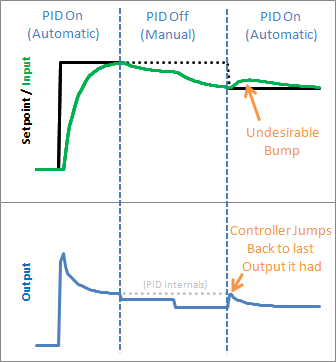Yikes! The PID jumps back to the last Output value it sent, then starts adjusting from there. This results in an Input bump that we’d rather not have.

### The Solution

This one is pretty easy to fix. Since we now know when we’re turning on (going from Manual to Automatic,) we just have to initialize things for a smooth transition. That means massaging the 2 stored working variables (ITerm & lastInput) to keep the output from jumping.

### The Code

```/*working variables*/
unsigned long lastTime;
double Input, Output, Setpoint;
double ITerm, lastInput;
double kp, ki, kd;
int SampleTime = 1000; //1 sec
double outMin, outMax;
bool inAuto = false;

#define MANUAL 0
#define AUTOMATIC 1

void Compute()
{
if(!inAuto) return;
unsigned long now = millis();
int timeChange = (now - lastTime);
if(timeChange>=SampleTime)
{
/*Compute all the working error variables*/
double error = Setpoint - Input;
ITerm+= (ki * error);
if(ITerm> outMax) ITerm= outMax;
else if(ITerm< outMin) ITerm= outMin;
double dInput = (Input - lastInput);

/*Compute PID Output*/
Output = kp * error + ITerm- kd * dInput;
if(Output> outMax) Output = outMax;
else if(Output < outMin) Output = outMin;

/*Remember some variables for next time*/
lastInput = Input;
lastTime = now;
}
}

void SetTunings(double Kp, double Ki, double Kd)
{
double SampleTimeInSec = ((double)SampleTime)/1000;
kp = Kp;
ki = Ki * SampleTimeInSec;
kd = Kd / SampleTimeInSec;
}

void SetSampleTime(int NewSampleTime)
{
if (NewSampleTime > 0)
{
double ratio  = (double)NewSampleTime
/ (double)SampleTime;
ki *= ratio;
kd /= ratio;
SampleTime = (unsigned long)NewSampleTime;
}
}

void SetOutputLimits(double Min, double Max)
{
if(Min > Max) return;
outMin = Min;
outMax = Max;

if(Output > outMax) Output = outMax;
else if(Output < outMin) Output = outMin;

if(ITerm> outMax) ITerm= outMax;
else if(ITerm< outMin) ITerm= outMin;
}

void SetMode(int Mode)
{
bool newAuto = (Mode == AUTOMATIC);
if(newAuto && !inAuto)
{  /*we just went from manual to auto*/
Initialize();
}
inAuto = newAuto;
}

void Initialize()
{
lastInput = Input;
ITerm = Output;
if(ITerm> outMax) ITerm= outMax;
else if(ITerm< outMin) ITerm= outMin;
}
```

We modified SetMode(…) to detect the transition from manual to automatic, and we added our initialization function. It sets ITerm=Output to take care of the integral term, and lastInput = Input to keep the derivative from spiking. The proportional term doesn’t rely on any information from the past, so it doesn’t need any initialization.

### The Result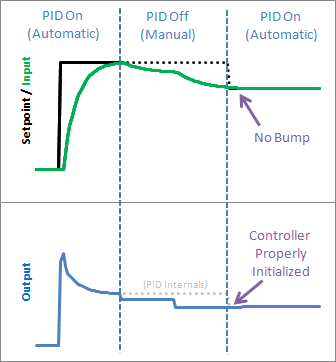We see from the above graph that proper initialization results in a bumpless transfer from manual to automatic: exactly what we were after.
Next >>

### Update: Why not ITerm=0?

I have been getting a lot of questions recently asking why I don’t set ITerm=0 upon intialization. As an answer, I’d ask you to consider the following scenario: The pid is in manual, and the user has set the output to 50. After a time, the process steadies out to an input of 75.2. The user makes the Setpoint 75.2 and turns on the pid. What should happen?

I contend that after switching to automatic the output value should stay at 50. since the P and D terms will be zero, the only way this will happen is if ITerm is initialized to the value of Output.

If you are in a situation where you need the output to initialize to zero, there is no need alter the code above. Just set Output=0 in your calling routine before turning the PID from Manual to Automatic.

### Improving the Beginner’s PID: On/Off

Friday, April 15th, 2011

(This is Modification #5 in a larger series on writing a solid PID algorithm)

### The Problem

As nice as it is to have a PID controller, sometimes you don’t care what it has to say.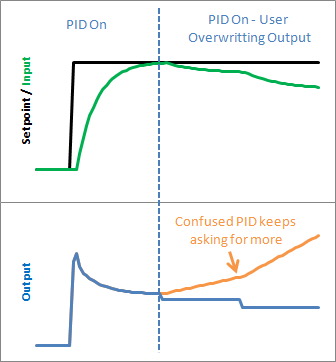Let’s say at some point in your program you want to force the output to a certain value (0 for example) you could certainly do this in the calling routine:

void loop()
{
Compute();
Output=0;
}

This way, no matter what the PID says, you just overwrite its value. This is a terrible idea in practice however. The PID will become very confused: “I keep moving the output, and nothing’s happening! What gives?! Let me move it some more.” As a result, when you stop over-writing the output and switch back to the PID, you will likely get a huge and immediate change in the output value.

### The Solution

The solution to this problem is to have a means to turn the PID off and on. The common terms for these states are “Manual” (I will adjust the value by hand) and “Automatic” (the PID will automatically adjust the output). Let’s see how this is done in code:

### The Code

```
/*working variables*/
unsigned long lastTime;
double Input, Output, Setpoint;
double ITerm, lastInput;
double kp, ki, kd;
int SampleTime = 1000; //1 sec
double outMin, outMax;
bool inAuto = false;

#define MANUAL 0
#define AUTOMATIC 1

void Compute()
{
if(!inAuto) return;
unsigned long now = millis();
int timeChange = (now - lastTime);
if(timeChange>=SampleTime)
{
/*Compute all the working error variables*/
double error = Setpoint - Input;
ITerm+= (ki * error);
if(ITerm> outMax) ITerm= outMax;
else if(ITerm< outMin) ITerm= outMin;
double dInput = (Input - lastInput);

/*Compute PID Output*/
Output = kp * error + ITerm- kd * dInput;
if(Output > outMax) Output = outMax;
else if(Output < outMin) Output = outMin;

/*Remember some variables for next time*/
lastInput = Input;
lastTime = now;
}
}

void SetTunings(double Kp, double Ki, double Kd)
{
double SampleTimeInSec = ((double)SampleTime)/1000;
kp = Kp;
ki = Ki * SampleTimeInSec;
kd = Kd / SampleTimeInSec;
}

void SetSampleTime(int NewSampleTime)
{
if (NewSampleTime > 0)
{
double ratio  = (double)NewSampleTime
/ (double)SampleTime;
ki *= ratio;
kd /= ratio;
SampleTime = (unsigned long)NewSampleTime;
}
}

void SetOutputLimits(double Min, double Max)
{
if(Min > Max) return;
outMin = Min;
outMax = Max;

if(Output > outMax) Output = outMax;
else if(Output < outMin) Output = outMin;

if(ITerm> outMax) ITerm= outMax;
else if(ITerm< outMin) ITerm= outMin;
}

void SetMode(int Mode)
{
inAuto = (Mode == AUTOMATIC);
}

```

A fairly simple solution. If you’re not in automatic mode, immediately leave the Compute function without adjusting the Output or any internal variables.

### The Result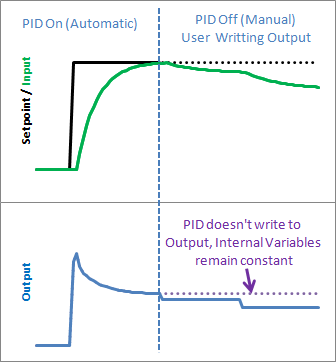It’s true that you could achieve a similar effect by just not calling Compute from the calling routine, but this solution keeps the workings of the PID contained, which is kind of what we need. By keeping things internal we can keep track of which mode were in, and more importantly it let’s us know when we change modes. That leads us to the next issue…
Next >>### Improving the Beginner’s PID: Reset Windup

Friday, April 15th, 2011

(This is Modification #4 in a larger series on writing a solid PID algorithm)

### The Problem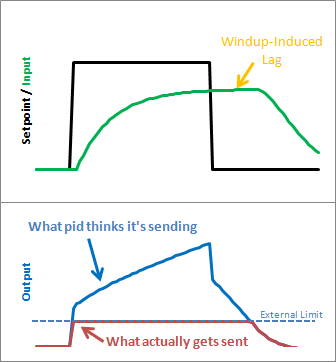Reset windup is a trap that probably claims more beginners than any other. It occurs when the PID thinks it can do something that it can’t. For example, the PWM output on an Arduino accepts values from 0-255. By default the PID doesn’t know this. If it thinks that 300-400-500 will work, it’s going to try those values expecting to get what it needs. Since in reality the value is clamped at 255 it’s just going to keep trying higher and higher numbers without getting anywhere.

The problem reveals itself in the form of weird lags. Above we can see that the output gets “wound up” WAY above the external limit. When the setpoint is dropped the output has to wind down before getting below that 255-line.

### The Solution – Step 1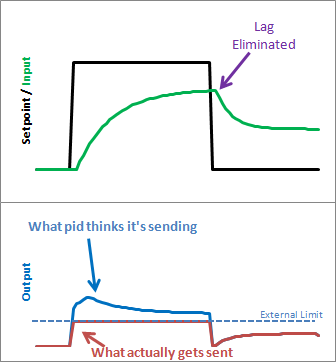There are several ways that windup can be mitigated, but the one that I chose was as follows: tell the PID what the output limits are. In the code below you’ll see there’s now a SetOuputLimits function. Once either limit is reached, the pid stops summing (integrating.) It knows there’s nothing to be done; Since the output doesn’t wind-up, we get an immediate response when the setpoint drops into a range where we can do something.

### The Solution – Step 2

Notice in the graph above though, that while we got rid that windup lag, we’re not all the way there. There’s still a difference between what the pid thinks it’s sending, and what’s being sent. Why? the Proportional Term and (to a lesser extent) the Derivative Term.

Even though the Integral Term has been safely clamped, P and D are still adding their two cents, yielding a result higher than the output limit. To my mind this is unacceptable. If the user calls a function called “SetOutputLimits” they’ve got to assume that that means “the output will stay within these values.” So for Step 2, we make that a valid assumption. In addition to clamping the I-Term, we clamp the Output value so that it stays where we’d expect it.

(Note: You might ask why we need to clamp both. If we’re going to do the output anyway, why clamp the Integral separately? If all we did was clamp the output, the Integral term would go back to growing and growing. Though the output would look nice during the step up, we’d see that telltale lag on the step down.)

### The Code

```/*working variables*/
unsigned long lastTime;
double Input, Output, Setpoint;
double ITerm, lastInput;
double kp, ki, kd;
int SampleTime = 1000; //1 sec
double outMin, outMax;
void Compute()
{
unsigned long now = millis();
int timeChange = (now - lastTime);
if(timeChange>=SampleTime)
{
/*Compute all the working error variables*/
double error = Setpoint - Input;
ITerm+= (ki * error);
if(ITerm> outMax) ITerm= outMax;
else if(ITerm< outMin) ITerm= outMin;
double dInput = (Input - lastInput);

/*Compute PID Output*/
Output = kp * error + ITerm- kd * dInput;
if(Output > outMax) Output = outMax;
else if(Output < outMin) Output = outMin;

/*Remember some variables for next time*/
lastInput = Input;
lastTime = now;
}
}

void SetTunings(double Kp, double Ki, double Kd)
{
double SampleTimeInSec = ((double)SampleTime)/1000;
kp = Kp;
ki = Ki * SampleTimeInSec;
kd = Kd / SampleTimeInSec;
}

void SetSampleTime(int NewSampleTime)
{
if (NewSampleTime > 0)
{
double ratio  = (double)NewSampleTime
/ (double)SampleTime;
ki *= ratio;
kd /= ratio;
SampleTime = (unsigned long)NewSampleTime;
}
}

void SetOutputLimits(double Min, double Max)
{
if(Min > Max) return;
outMin = Min;
outMax = Max;

if(Output > outMax) Output = outMax;
else if(Output < outMin) Output = outMin;

if(ITerm> outMax) ITerm= outMax;
else if(ITerm< outMin) ITerm= outMin;
}
```

A new function was added to allow the user to specify the output limits [lines 52-63]. And these limits are used to clamp both the I-Term [17-18] and the Output [23-24]

### The Result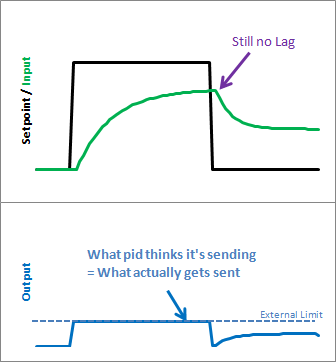As we can see, windup is eliminated. in addition, the output stays where we want it to. this means there’s no need for external clamping of the output. if you want it to range from 23 to 167, you can set those as the Output Limits.
Next >>### Improving the Beginner’s PID: Tuning Changes

Friday, April 15th, 2011

(This is Modification #3 in a larger series on writing a solid PID algorithm)

### The Problem

The ability to change tuning parameters while the system is running is a must for any respectable PID algorithm.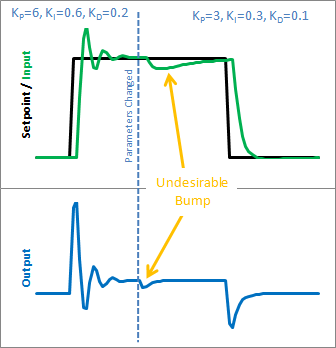The Beginner’s PID acts a little crazy if you try to change the tunings while it’s running. Let’s see why. Here is the state of the beginner’s PID before and after the parameter change above: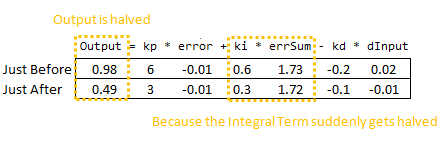So we can immediately blame this bump on the Integral Term (or “I Term”). It’s the only thing that changes drastically when the parameters change. Why did this happen? It has to do with the beginner’s interpretation of the Integral: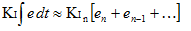This interpretation works fine until the Ki is changed. Then, all of a sudden, you multiply this new Ki times the entire error sum that you have accumulated. That’s not what we wanted! We only wanted to affect things moving forward!

### The Solution

There are a couple ways I know of to deal with this problem. The method I used in the last library was to rescale errSum. Ki doubled? Cut errSum in Half. That keeps the I Term from bumping, and it works. It’s kind of clunky though, and I’ve come up with something more elegant. (There’s no way I’m the first to have thought of this, but I did think of it on my own. That counts damnit!)

The solution requires a little basic algebra (or is it calculus?)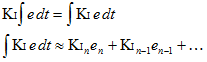Instead of having the Ki live outside the integral, we bring it inside. It looks like we haven’t done anything, but we’ll see that in practice this makes a big difference.

Now, we take the error and multiply it by whatever the Ki is at that time. We then store the sum of THAT. When the Ki changes, there’s no bump because all the old Ki’s are already “in the bank” so to speak. We get a smooth transfer with no additional math operations. It may make me a geek but I think that’s pretty sexy.

### The Code

```/*working variables*/
unsigned long lastTime;
double Input, Output, Setpoint;
double ITerm, lastInput;
double kp, ki, kd;
int SampleTime = 1000; //1 sec
void Compute()
{
unsigned long now = millis();
int timeChange = (now - lastTime);
if(timeChange>=SampleTime)
{
/*Compute all the working error variables*/
double error = Setpoint - Input;
ITerm += (ki * error);
double dInput = (Input - lastInput);

/*Compute PID Output*/
Output = kp * error + ITerm - kd * dInput;

/*Remember some variables for next time*/
lastInput = Input;
lastTime = now;
}
}

void SetTunings(double Kp, double Ki, double Kd)
{
double SampleTimeInSec = ((double)SampleTime)/1000;
kp = Kp;
ki = Ki * SampleTimeInSec;
kd = Kd / SampleTimeInSec;
}

void SetSampleTime(int NewSampleTime)
{
if (NewSampleTime > 0)
{
double ratio  = (double)NewSampleTime
/ (double)SampleTime;
ki *= ratio;
kd /= ratio;
SampleTime = (unsigned long)NewSampleTime;
}
}

```

So we replaced the errSum variable with a composite ITerm variable [Line 4]. It sums Ki*error, rather than just error [Line 15]. Also, because Ki is now buried in ITerm, it’s removed from the main PID calculation [Line 19].

### The Result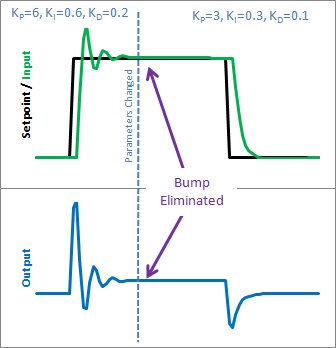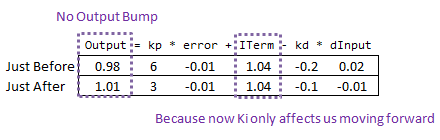So how does this fix things. Before when ki was changed, it rescaled the entire sum of the error; every error value we had seen. With this code, the previous error remains untouched, and the new ki only affects things moving forward, which is exactly what we want.
Next >>### Improving the Beginner’s PID – Derivative Kick

Friday, April 15th, 2011

(This is Modification #2 in a larger series on writing a solid PID algorithm)

### The Problem

This modification is going to tweak the derivative term a bit. The goal is to eliminate a phenomenon known as “Derivative Kick”.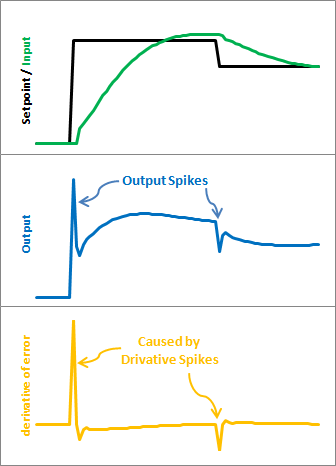The image above illustrates the problem. Since error=Setpoint-Input, any change in Setpoint causes an instantaneous change in error. The derivative of this change is infinity (in practice, since dt isn’t 0 it just winds up being a really big number.) This number gets fed into the pid equation, which results in an undesirable spike in the output. Luckily there is an easy way to get rid of this.

### The Solution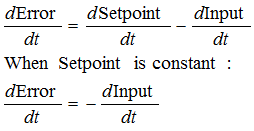It turns out that the derivative of the Error is equal to negative derivative of Input, EXCEPT when the Setpoint is changing. This winds up being a perfect solution. Instead of adding (Kd * derivative of Error), we subtract (Kd * derivative of Input). This is known as using “Derivative on Measurement”

### The Code

```/*working variables*/
unsigned long lastTime;
double Input, Output, Setpoint;
double errSum, lastInput;
double kp, ki, kd;
int SampleTime = 1000; //1 sec
void Compute()
{
unsigned long now = millis();
int timeChange = (now - lastTime);
if(timeChange>=SampleTime)
{
/*Compute all the working error variables*/
double error = Setpoint - Input;
errSum += error;
double dInput = (Input - lastInput);

/*Compute PID Output*/
Output = kp * error + ki * errSum - kd * dInput;

/*Remember some variables for next time*/
lastInput = Input;
lastTime = now;
}
}

void SetTunings(double Kp, double Ki, double Kd)
{
double SampleTimeInSec = ((double)SampleTime)/1000;
kp = Kp;
ki = Ki * SampleTimeInSec;
kd = Kd / SampleTimeInSec;
}

void SetSampleTime(int NewSampleTime)
{
if (NewSampleTime > 0)
{
double ratio  = (double)NewSampleTime
/ (double)SampleTime;
ki *= ratio;
kd /= ratio;
SampleTime = (unsigned long)NewSampleTime;
}
}
```

The modifications here are pretty easy. We’re replacing +dError with -dInput. Instead of remembering the lastError, we now remember the lastInput

### The Result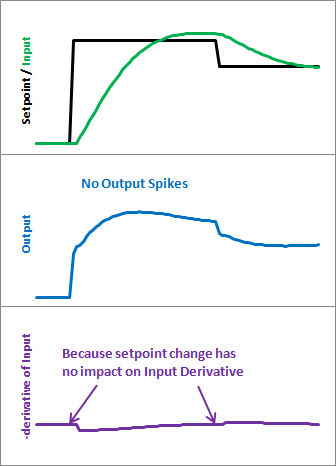Here’s what those modifications get us. Notice that the input still looks about the same. So we get the same performance, but we don’t send out a huge Output spike every time the Setpoint changes.

This may or may not be a big deal. It all depends on how sensitive your application is to output spikes. The way I see it though, it doesn’t take any more work to do it without kicking so why not do things right?
Next >>